Search by Topic

Filter by: Content type:
Age range:
Challenge level:

There are 25 results

Broad Topics > Measuring and calculating with units > Surface and surface area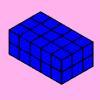Changing Areas, Changing Volumes

Age 11 to 14 Challenge Level:

How can you change the surface area of a cuboid but keep its volume the same? How can you change the volume but keep the surface area the same?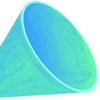Funnel

Age 14 to 16 Challenge Level:

A plastic funnel is used to pour liquids through narrow apertures. What shape funnel would use the least amount of plastic to manufacture for any specific volume ?Cola Can

Age 11 to 14 Challenge Level:

An aluminium can contains 330 ml of cola. If the can's diameter is 6 cm what is the can's height?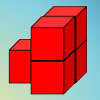Age 7 to 11 Challenge Level:

How can you arrange the 5 cubes so that you need the smallest number of Brush Loads of paint to cover them? Try with other numbers of cubes as well.Cuboids

Age 11 to 14 Challenge Level:

Find a cuboid (with edges of integer values) that has a surface area of exactly 100 square units. Is there more than one? Can you find them all?The Spider and the Fly

Age 14 to 16 Challenge Level:

A spider is sitting in the middle of one of the smallest walls in a room and a fly is resting beside the window. What is the shortest distance the spider would have to crawl to catch the fly?Painted Cube

Age 14 to 16 Challenge Level:

Imagine a large cube made from small red cubes being dropped into a pot of yellow paint. How many of the small cubes will have yellow paint on their faces?Three Cubes

Age 14 to 16 Challenge Level:

Can you work out the dimensions of the three cubes?Cubes

Age 7 to 11 Challenge Level:

How many faces can you see when you arrange these three cubes in different ways?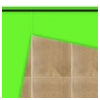Tiling Into Slanted Rectangles

Age 7 to 11 Challenge Level:

A follow-up activity to Tiles in the Garden.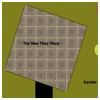Tiles in the Garden

Age 7 to 11 Challenge Level:

How many tiles do we need to tile these patios?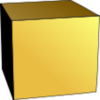Uniform Units

Age 14 to 16 Challenge Level:

Can you choose your units so that a cube has the same numerical value for it volume, surface area and total edge length?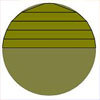Peeling the Apple or the Cone That Lost Its Head

Age 14 to 16 Challenge Level:

How much peel does an apple have?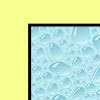All Wrapped Up

Age 7 to 11 Challenge Level:

What is the largest cuboid you can wrap in an A3 sheet of paper?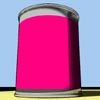Tin Tight

Age 14 to 16 Challenge Level:

What's the most efficient proportion for a 1 litre tin of paint?Inside Out

Age 14 to 16 Challenge Level:

There are 27 small cubes in a 3 x 3 x 3 cube, 54 faces being visible at any one time. Is it possible to reorganise these cubes so that by dipping the large cube into a pot of paint three times you. . . .All Tied Up

Age 14 to 16 Challenge Level:

A ribbon runs around a box so that it makes a complete loop with two parallel pieces of ribbon on the top. How long will the ribbon be?When the Angles of a Triangle Don't Add up to 180 Degrees

Age 14 to 18

This article outlines the underlying axioms of spherical geometry giving a simple proof that the sum of the angles of a triangle on the surface of a unit sphere is equal to pi plus the area of the. . . .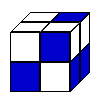Cubic Conundrum

Age 7 to 16 Challenge Level:

Which of the following cubes can be made from these nets?Take Ten

Age 11 to 14 Challenge Level:

Is it possible to remove ten unit cubes from a 3 by 3 by 3 cube so that the surface area of the remaining solid is the same as the surface area of the original?Plutarch's Boxes

Age 11 to 14 Challenge Level:

According to Plutarch, the Greeks found all the rectangles with integer sides, whose areas are equal to their perimeters. Can you find them? What rectangular boxes, with integer sides, have. . . .F'arc'tion

Age 14 to 16 Short Challenge Level:

At the corner of the cube circular arcs are drawn and the area enclosed shaded. What fraction of the surface area of the cube is shaded? Try working out the answer without recourse to pencil and. . . .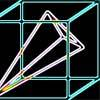Four Points on a Cube

Age 16 to 18 Challenge Level:

What is the surface area of the tetrahedron with one vertex at O the vertex of a unit cube and the other vertices at the centres of the faces of the cube not containing O?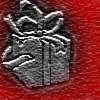Wrapping Presents

Age 7 to 11 Challenge Level:

Choose a box and work out the smallest rectangle of paper needed to wrap it so that it is completely covered.The Big Cheese

Age 7 to 11 Challenge Level:

Investigate the area of 'slices' cut off this cube of cheese. What would happen if you had different-sized block of cheese to start with?